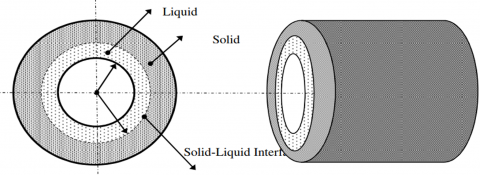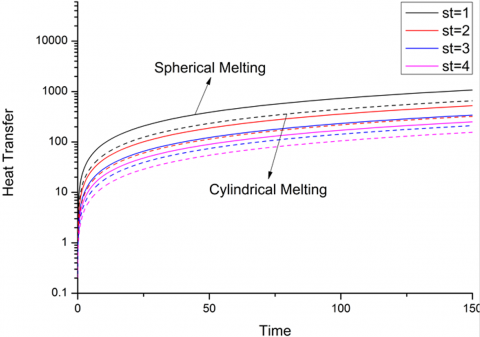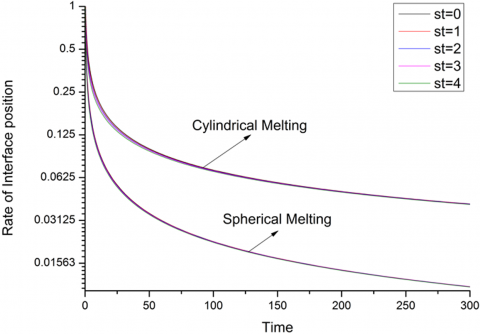# Mathematical Modelling for the Performance of Encapsulated Phase Change TESS and Effect of Stefan’s Number

Mathematical Modelling for the Performance of Encapsulated Phase Change TESS and Effect of Stefan’s Number
Department of Mechanical Engineering, NIT Jamshedpur-831014, India

Corresponding Author Email:
mayank.nitjamshedpur@gmail.com
Page:
280-284
|
DOI:
https://doi.org/10.18280/mmep.060216
21 August 2018
|
Accepted:
14 November 2018
|
Published:
20 June 2019
| Citation

OPEN ACCESS

Abstract:

Thermal energy storage system with phase change materials become increasingly important topics because of its important role in latent heat energy conservation, and for heating and cooling purpose. Thermal energy storage provides a great solution for the mismatch between energy production and its demand. TESS gives a high thermal storage density with a wide range of temperature. This paper considers the numerical solution of outward melting/solidification of encapsulated phase change materials in thermal energy storage system performance. Due to its nonlinear behaviour, it is complicated to have exact solution of melting process. HBI method is applied to solve uni-directional outward melting problem in cylindrical and spherical geometries. Interface location, heat transfer rate and heat transfer with time is obtained for both the geometries. A Matlab code has been written to solve moving interface problem.

Keywords:

conduction, encapsulation, HBI method, interface movement, melting/solidification nonlinear behavior, phase change materials, Stefan’s number

1. Introduction

The continuous depletion of non-renewable energy raises serious issues of their future availability and effect on environment. The increasing level of greenhouse gas emissions is the main reason behind the need of safe and clean energy alternative. Sun is the main source of clean and safe energy but there is a huge difference between its demand and supply because of its oscillating nature. Solar light is only available at day time, due to this fluctuation, we need a device which store renewable energy at faster rate and release whenever needed. This can be done by the use of thermal energy storage system (TESS). TESS used to store thermal energy as a form of sensible heat or latent heat. In Sensible thermal energy storage system, thermal energy is stored by increasing the temperature of storage medium while in Latent thermal energy storage system, thermal energy is stored at constant temperature.

Phase change materials used in TESS are materials, which store thermal energy due to its large heat storage capacity at constant temperature. PCM are broadly categorised into 3 main parts: organic, inorganic and eutectic . The choice of PCM is totally based on their melting temperature and its applications.

All the other materials which melt in between these two temperature limit are used in heating purpose . A technique which is used to keep the PCM in a different geometrical container to avoid any direct contact between the PCM and HTF is known as encapsulation. The size and shape of the PCM encapsulation is important to ensure long-term thermal performance of any thermal energy storage system and also the melting / solidification time of the PCM. There are some specific geometries, which commonly employed as PCM encapsulation are the rectangular, cylindrical and spherical encapsulation .

There are a series of experimental and analytical investigation has been done by the researchers on the phase change thermal energy storage system of different geometry under different boundary conditions. A phase change problem should be solved separately due to its non-linear nature of the problem. There are a wide range of different methods like Heat balance integral method [7, 13, 16], enthalpy method [13, 16] and finite difference methods  available for solving non-linear phase change problems. Melting and freezing problem of PCM in thermal energy storage system solved by HBIM and its accuracy is improved by subdividing dependent variable into equal intervals by G.E.Bell . A system of first order, non-linear differential equations has been produced to calculate the position of each isotherm. The location and time history of the 1-dimensional solid-liquid interface during the solidification of semi-infinite, cylindrical and spherical geometries has been investigated by G.Poots  by approximate integral method, which is very much similar to the solution of the boundary layer equations. Anant Prasad  has been numerically analysed the semi-infinite solid of constant cross section area under 1-dimensional convective heating to calculate the temperature build-up, thermal penetration depth and melt fraction by using Biot variational method.

Several researchers continuously tried to improve the accuracy of non-linear phase change thermal energy storage problem. The accuracy of HBIM has been considerably improved by successive sub division of the dependent variable [4, 7] and / or by choosing suitable temperature profile [6, 9, 13]. Some alternative ways to develop the original quadratic HBI has been given by A.S.Wood  to solve melting of semi-infinite slab which is initially at its melting temperature. T.G.Myers  developed a new method to find out the best possible value of power of highest order term in the approximating function used in HBIM by minimizing the square of the difference of the terms in the heat equations.

James Caldwell & C.K.Chiu  investigated solidification problem of PCM in spherical encapsulated phase change thermal energy storage system by using front tracking method and found that in spherical case the solidification front moves slightly slower. A.Kumar et al.  solved spherical outward melting of PCM in radial direction by employing Variational, Integral and Quasi-steady method. He-Sheng Ren  has solved the 1-dimensional inward solidification in Cartesian and spherical encapsulation problem by heat balance method. The melting phenomenon of PCM, encapsulated in a rectangular geometry under radiative heat injection has been analysed by A.Prasad et al.  by biot variational method, HBIM & quasi-static method. The results obtained by all these methods were almost same. R.K.Sharma et al.  obtained the solution of a 2-dimensional solidification problem in isosceles trapezoidal cavity by using CFD software and found that the heat transfer mainly occurs due to the conduction. Explicit finite difference method has been applied by K.Morgan  to solve freezing and melting phenomenon in a cylindrical thermal cavity. The combined effect of conduction and convection heat transfer was considered by this method and validated this numerical result with experimental result.

A modified variable time step method has been obtained by R.S.Gupta and Dhirendra Kumar  to solve solidification problem of liquid which is initially at its fusion temperature. The result obtained for the movement of the interface, temperature distribution and compared results with the result found by HBI method. James Caldwell and Ching-chuen Chan  numerically analysed PCM solidification by enthalpy method & HBIM over a wide range of the Stefan number except for very small value. The shell and tube type latent heat thermal energy storage system during melting and solidification has been numerically and experimentally analysed by Anica Trp  to evaluate heat transfer during process.

Dinu G Thomas et al.  experimentally analysed energy and exergy analysis of PCM during melting process. Sodium thiosulfate pentahydrate used as PCM in this analysis. Piia Lamberg et al.  numerically and experimentally investigated the PCM energy storage device with and without heat transfer enhancement structure by enthalpy method and heat capacity method. The results obtained then compared with experimental results. Finite difference approach has been used by Stetislav Savovic et al.  to analyse the 1-dimensional Stefan problem with periodic fixed boundary condition.

In this present paper, the mathematical modelling of phase change thermal energy storage system has been formulated for charging process. The behaviour of PCM melting in a cylindrical and spherical encapsulation under fixed boundary is being analysed by using MATLAB coding. The effect of Stefan number on dimensionless interface location, heat transfer and rate of interface position is discussed.

2. Mathematical Modelling

In the present study, a schematic drawing of a spherical and cylindrical geometry containing solid PCM used is shown in Figure.1. We consider a single phase, 1-dimensional outward melting problem of PCM kept inside a spherical and cylindrical capsule, while in practical, the melting problem is rarely one dimensional, initial and boundary conditions are always complex. Initially, PCM is at its melting temperature Tm. The temperature at the geometry boundary is TS, which is higher than the PCM melting temperature Tm.

The term ‘moving boundary problems’ is associated with time-dependent boundary problems and also referred as Stefan problems, where the position of the moving boundary must be determined as a function of time and space. As the time passes solid PCM will melt due to the boundary temperature applied at vessel and the governing equations for this process may be described by:

$\frac{1}{r^{k}} \cdot \frac{\partial}{\partial r}\left[r^{k} \cdot \frac{\partial T}{\partial r}\right]=\cdot \frac{1}{\alpha} \cdot \frac{\partial T}{\partial t}$ (1)

where k =1   for cylindrical geometry

k =2   for spherical geometry

## 1.pngFigure 1. Schematic diagram of encapsulated PCM inside cylindrical geometry undergoing outward melting

Boundary Conditions are,

r = ri ,       t > 0,       T = Ts (2)

r = δ ,       t > 0,       T = T(3)

r  ≥ ri,       t = 0,       T = Tm (4)

Energy balance equation at the solid-liquid interface is,

$\left[K \cdot \frac{\partial T}{\partial x}\right]=-\rho L \cdot \frac{\partial \delta}{\partial t}$ (5)

To reduce dependent variables we introduce the non dimensional variables,

$\xi=\frac{r}{r_{i}}$, $\eta=\frac{\delta}{r_{i}}$, $\tau=\frac{\alpha t}{r_{i}^{2}}$, $\theta=\frac{T-T_{f}}{T_{s}-T_{f}}$, $S_{t}=\frac{C\left(T_{s}-T_{f}\right)}{l}$

Now governing Eq. (1) can be written as,

$\frac{1}{\xi^{k}} \frac{\partial}{\partial \xi}\left[\xi^{k} \frac{\partial \theta}{\partial \xi}\right]=\frac{\partial \theta}{\partial \tau}$ (6)

And all boundary conditions become,

$\xi=1, \qquad \tau>0, \qquad \theta=1$ (7)

$\xi=\eta, \quad \tau>0, \quad \theta=0$ (8)

$\xi \geq 1, \quad \tau=0_{2} \quad \theta=0$ (9)

Now non-dimensional energy balance equation at the solid-liquid interface is,

$\left[\frac{\partial \theta}{\partial \xi}\right]=-\frac{1}{S_{t}} \frac{\partial \eta}{\partial \tau}$ (10)

2.1 Heat balance integral method

The heat balance integral method (HBIM) is simple approximate technique developed for solving transport problems like phase change problems. Goodman  introduced HBIM, which converts the governing partial differential equations to ordinary differential equations by:

1. Assuming the most suitable approximate temperature profile, either linear, quadratic, cubic, exponential etc.
2. Satisfying the boundary conditions,
3. Integrate the heat conduction equation with respect to the space variable over a suitable interval to create a heat balance integral equation.
4. Solve the integral equation to obtain the interface location and temperature distribution.

Now, integrate the energy equation (10) with respect to space variable,

$\int_{1}^{\eta} \frac{\partial}{\partial \xi}\left[\xi^{k} \frac{\partial \theta}{\partial \xi}\right] d \xi=\int_{1}^{\eta}\left[\xi^{k} \frac{\partial \theta}{\partial \tau}\right] d \xi$ (11)

\begin{aligned} \frac{\partial}{\partial \tau}\left[\int_{1}^{\eta}\left(\xi^{k} \theta\right) d \xi\right] &-\left(\xi^{k} \theta\right)_{\xi=\eta} \dot{\eta} \\ &=\left[\xi^{k} \frac{\partial \theta}{\partial \xi}\right]_{\xi=\eta}-\left[\xi^{k} \frac{\partial \theta}{\partial \xi}\right]_{\xi=1} \end{aligned} (12)

Now assume a suitable linear temperature profile with negligible temperature drop within the solid layer, which satisfies the boundary conditions:

$\theta=1-\left[\begin{array}{c}{1-\frac{1}{\xi}} \\ {1-\frac{1}{\eta}}\end{array}\right]$ (13)

2.2 Interface location analysis

Substituting Eq. (13) into Eq. (12) leads to, for spherical geometry (k=2),

$\tau=\frac{\eta^{3}}{3}-\frac{\eta^{2}}{2}-\frac{1}{6}+\left[\eta^{2}-\log (\eta)-\eta\right]\left(\frac{S_{t}}{6}\right)$ (14)

For cylindrical geometry (k=1),

$\tau=\frac{\eta^{2}}{3}-\eta+\frac{1}{2}+[\eta-\log (\eta)-1]\left(\frac{S_{t}}{2}\right)$ (15)

2.3 Heat transfer analysis

$\mathrm{Q}=\int_{1}^{\mathrm{\eta}}(\text { Latent heat }+\text { Sensible heat }) \mathrm{d} \xi$

For sphere:

$\mathrm{Q}_{\tau}=\left[\frac{\eta^{3}-1}{S_{\mathrm{t}}}+3 \int_{1}^{\eta} \xi^{2} \theta \mathrm{d} \xi\right]$ (16)

Substituting Eq. (13) into Eq. (16) leads to

\begin{aligned} Q_{\tau}=\left[\frac{\eta^{3}-1}{S_{t}}+\left(\eta^{3}-1\right)\right.& \\ &-3\left(\frac{\eta}{\eta-1}\right)\left(\frac{\eta^{3}-1}{3}-\frac{\eta^{2}-1}{2}\right) ] \end{aligned} (17)

For cylinder:

$Q_{\tau}=\left[\frac{\eta^{2}-1}{S_{t}}+2 \int_{1}^{\eta} \xi \theta d \xi\right]$ (18)

Substituting Eq. (13) into Eq. (18) leads to

\begin{aligned} \mathrm{Q}_{\mathrm{r}}=\left[\frac{\eta^{3}-1}{\mathrm{S}_{\mathrm{t}}}+\left(\eta^{3}-1\right)\right.& \\ &-3\left(\frac{\eta}{\eta-1}\right)\left(\frac{\eta^{3}-1}{3}-\frac{\eta^{2}-1}{2}\right) ] \end{aligned} (19)

3. Results and Discussion

In this part we present the numerical results obtained by using heat balance integral method in cylindrical and spherical melting process of phase change materials used in thermal energy storage.

## 2.pngFigure 2. Behaviour of the non-dimensional interface location with non-dimensional time, Stefan’s number is taken as a parameter

Figure 2 shows the variation of dimensionless interface location or interface depth with time for different values of Stefan’s number. With each values of Stefan number, during starting time of melting, the interface depth increases very rapidly with time and as the time passes, interface depth becomes almost linear with time because during starting time period heat transfer rate increases more quickly. By using HBIM the melting process of PCM used in latent heat thermal energy storage system is strongly depends upon Stefan’s number. At any time instance interface depth with time decreases, as Stefan’s number decreases.

## 3.pngFigure 3. Non-dimensional heat transfer with non-dimensional time, Stefan’s number is taken as a parameter

The variation of dimensionless heat transfer with time in spherical and cylindrical melting for different values of Stefan’s number is shown in Figure 3. During initial time of melting of PCM, the heat transfer increases more rapidly because of large temperature difference available between two phases. But with time, heat transfer becomes linear because the temperate difference between two phases decreases. This means that, except the initial time period, the heat transfer process is very slow. The time taken to absorb a certain amount of heat in cylindrical process is higher than that of spherical melting process.

The rate of interface depth or the interface velocity is a function of Stefan’s number, as seen in equation 14 & 16. The variation of interface velocity with time is shown in Figure 4. The rate of interface position, which is a single curve, decreases with time, for all values of Stefan’s number.

## 4.pngFigure 4. Behaviour of the non-dimensional rate interface position with non-dimensional time, Stefan’s number is taken as a parameter

4. Conclusions

The mathematical modelling for melting phenomenon of PCM at constant temperature heat transfer in cylindrical and spherical encapsulated thermal energy system has been formulated and solved by heat balance integral method for interface depth, heat transfer and interface velocity. In present work, HBIM is used only for single phase change model. This method can be further applied on more realistic melting or solidification problems. The heat transfer process between two phases occurs only by conduction. Convection and radiation heat transfer is being neglected in this work.

Nomenclature
 C Heat capacity k constant, k=1,2 for cylinder & sphere respectively K thermal conductivity, W/m K L latent heat,  J/Kg Qτ non-dimensional total heat absorbed R radius, m ri inner radius, m St Stefan number, $\frac{C\left(T_{s}-T_{m}\right)}{L}$ t time, s Tm melting temperature, K Ts surface temperature, K Greek symbols a thermal diffusivity, m2/s δ Interface location ƞ non-dimensional radial distance of phase front, δ/ri $\dot{\eta}$ time rate of non-dimensional radial distance of phase front, d ƞ/d τ ξ non-dimensional radial distance within phase change, r/ri ρ density, Kg/m3 θ non-dimensional temperature,  $\frac{T-T_{m}}{T_{s}-T_{m}}$ τ non-dimensional time, $\frac{\alpha t}{r_{i}^{2}}$
References

 Zalba B, Ma Marın J, Cabeza LF, Mehling H. (2003). Review on thermal energy storage with phase change: Materials, heat transfer analysis and applications. Applied Thermal Engineering 23: 251–283. http://dx.doi.org/10.1016/S1359-4311(02)00192-8

 Farid MM., Khudhair AM, Razack SAK., AI-Hallaj S. (2004). A review on phase change energy storage: materials and applications. Energy Conversion and Management 45: 1597–1615. http://dx.doi.org/10.1016/j.enconman.2003.09.015

 Agyenim F, Hewitt N, Eames P, Smyth M. (2010). A review of materials, heat transfer and phase change problem formulation for latent heat thermal energy storage systems (LHTESS). Renewable and Sustainable Energy Reviews 14: 615–628. http://dx.doi.org/10.1016/j.rser.2009.10.015

 Bell GE. (1978). A refinement of the heat balance integral method applied to a melting problem. Int. J. Heat Mass Transfer 21: 1357-1362.

 Poots G. (1962). On the application of integral methods to the solution of the problem involving the solidification of liquids initially at fusion temperature. Int. J. Heat Mass Transfer 15: 525-531. http://dx.doi.org/10.1016/0017-9310(62)90163-1

 Prasad A. (1979). Melting of solid bodies due to convective heating with the removal of melt. American Institute of Aeronautics and Astronautics, Inc. 16: 445-448.

 Caldwell J, Chiu CK. (2000). Numerical solution of one phase Stefan problems BT the heat balance integral method, Past-I – cylindrical and spherical geometries. Communications in Numerical Methods in Engineering 16: 569-583.

 Kumar A, Prasad A, Upadhaya SN. (1987). Spherical Phase change energy storage with constant temperature heat injection. Journal of Energy Resources Technology 109: 101-104. http://dx.doi.org/10.1115/1.3231333

 Ren HS. (2007). Application of the heat balance integral to an inverse Stefan problem. Int. J. of Thermal Sciences 46: 118-127. http://dx.doi.org/10.1016/j.ijthermalsci.2006.04.013

 Prasad A, Singh SP. (1994). Conduction controlled phase change energy storage with radiative heat addition. Transactions of the ASME 116: 218-223.

 Sharma RK, Ganesan P, Sahu JN, Metselaar HSC, Mahila TMI. (2014). Numerical study for enhancement of solidification of phase change materials using trapezoidal cavity. Int. J. of Powder Technology 268: 38-47. http://dx.doi.org/10.1016/j.powtec.2014.08.009

 Morgan K. (1981). A numerical analysis of freezing and melting with convection. Computer Methods in Applied Mechanics and Engineering 28: 275-284. http://dx.doi.org/10.1016/0045-7825(81)90002-5

 Wood AS. (2001). A new look at the heat balance integral method. Applied Mathematical Modelling 25: 818-824.

 Myers TG, Mitchell SL, Muchatibaya G, Myers MY. (2007). A cubic heat balance integral method for one dimensional melting of a finite thickness layer. Int. J. Heat Mass Transfer 50: 5305-5317. http://dx.doi.org/10.1016/j.ijheatmasstransfer.2007.06.014

 Gupta RS, Kumar D. (1981). Variable time step methods for one-dimensional Stefan problem with mixed boundary condition. Int. J. Heat Mass Transfer 24: 251-259. http://dx.doi.org/10.1016/0017-9310(81)90033-8

 Caldwell J, Chan CCH. (2000). Spherical solidification by the enthalpy method and the heat balance integral method. Applied Mathematical Modelling 24: 45-53. http://dx.doi.org/10.1016/S0307-904X(99)00031-1

 Trp A. (2005). An experimental and numerical investigation of heat transfer during technical grade paraffin melting and solidification in a shell and tube latent thermal energy storage unit. Int. J. of Solar Energy 79: 648-660. http://dx.doi.org/10.1016/j.solener.2005.03.006

 Dinu G. Thomas, Sajith Babu C, Sajith Gopi (2016). Performance analysis of a latent heat thermal energy storage system for solar energy applications. Inernational Conferrence on Emerging Trends in Engineering, Science and Technology 24: 469-476.

 Lamberg P, Lehtiniemi R, Henell AM. (2004). Numerical and experimental investigation of melting and freezing processes in phase change material storage. Int. J. of Thermal Sciences 43: 277-287. http://dx.doi.org/10.1016/j.ijthermalsci.2003.07.001

 Savovic S, Caldwell J. (2009). Numerical solution of Stefan problem with time-dependent boundary conditions by variable space grid method. Int. J. of Thermal Science 13: 165-174. http://dx.doi.org/10.2298/TSCI0904165S

 Goodman TR. (1958). The heat balance integral and its application to problems involving a change of phase. Trans. ASME Journal of Heat Transfer 80: 335-342.

 Srivastava M, Sinha MK. (2018). Mathematical analysis of phase change thermal energy storage system and effect of Stefan’S number on TESS performance. Advances in Modelling and Analysis A 55(4): 217-221. https://doi.org/10.18280.ama_a.550406

 Trancossi M, Pascoa J. (2018). A new dimensionless approach to general fluid dynamics problems that accounts both the first and the second law of thermodynamics. Mathematical Modelling and Engineering Problems 5(4): 331-340. https://doi.org/10.18280/mmep.050409